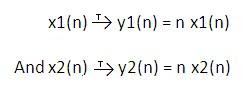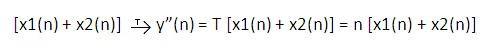# Linear or Non-linear Systems (Linearity Property)

## Linear or Non-linear Systems (Linearity Property):

A linear system is a system which follows the superposition principle. Let us consider a system having its response as ‘T’, input as x(n) and it produces output y(n). This is shown in figure below:

Let us consider two inputs. Input x1(n) produces output y1(n) and input x2(n) produces output y2(n). Now consider two arbitrary constants a1 and a2. Then simply multiply these constants with input x1(n) and x2(n) respectively. Thus a1x1(n) produces output a1y1(n) and a2x2(n) produces output a2y2(n).

### Theorem for linearity of the system:

A system is said to be linear if the combined response of a1x1(n) and a2x2(n) is equal to the addition of the individual responses.

That means,

T[a1 x1(n) + a2 x2(n)] = a1 T[x1(n)] + a2 T[x2(n)]…………….1)

The above theorem is also known as superposition theorem.

Important Characteristic:

Linear system has one important characteristic: If the input to the system is zero then it produces zero output. If the given system produces some output (non-zero) at zero input then the system is said to be Non-linear system. If this condition is satisfied then apply the superposition theorem to determine whether the given system is linear or not?

For continuous time system:

Similar to the discrete time system a continuous time system is said to be linear if it follows the superposition theorem.

Let us consider two systems as follows:

y1(t) = f[x1(t)]

And y2(t) = f[x2(t)]

Here y1(t) and y2(t) are the responses of the system and x1(t) and x2(t) are the excitations. Then the system is said to be linear if it satisfies the following expression:

f[a1 x1(t) + a2 x2(t)] = a1 y1(t) + a2 y2(t)…………….1)

Where a1 and a2 are constants.

A system is said to be non-linear system if does not satisfies the above expression. Communication channels and filters are examples of linear systems.

How to determine whether the given system is Linear or not?

To determine whether the given system is Linear or not, we have to follow the following steps:

Step 1: Apply zero input and check the output. If the output is zero then the system is linear. If this step is satisfied then follow the remaining steps.

Step 2: Apply individual inputs to the system and determine corresponding outputs. Then add all outputs. Denote this addition by y’(n). This is the R.H.S. of the 1st equation.

Step 3: Combine all inputs. Apply it to the system and find out y”(n). This is L.H.S. of equation (1).

Step 4: if y’(n) = y”(n) then the system is linear otherwise it is non-linear system.

### Solved problem:

Determine whether the following system is linear or not?

y(n) = n x(n)

Solution:

Step 1: When input x(n) is zero then output is also zero. Here first step is satisfied so we will check remaining steps for linearity.

Step 2: Let us consider two inputs x1(n) and x2(n) be the two inputs which produces outputs y1(t) and y2(t) respectively. It is given as follows:Now add these two output to get y’(n)

Therefore y’(n) = y1(n) + y2(n) = n x1(n) + n x2(n)

Therefore y’(n) = n [x1(n) + x2(n)]

Step 3: Now add x1(n) and x2(n) and apply this input to the system.

ThereforeWe know that the function of system is to multiply input by ‘n’.

Here [x1(n) + x2(n)] acts as one input to the system. So the corresponding output is,

y”(n) = n [x1(n) + x2(n)]

Step 4: Compare y’(n) and y”(n).

Here y’(n) = y”(n). hence the given system is linear.

### You may also like:

[contact-form][contact-field label='Name' type='name' required='1'/][contact-field label='Email' type='email' required='1'/][contact-field label='Website' type='url'/][contact-field label='Comment' type='textarea' required='1'/][/contact-form]1.